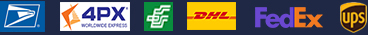•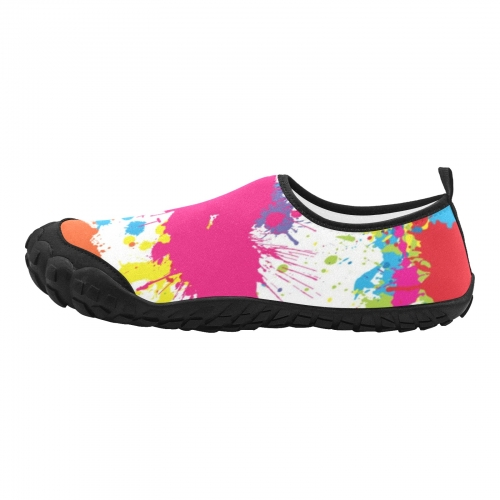•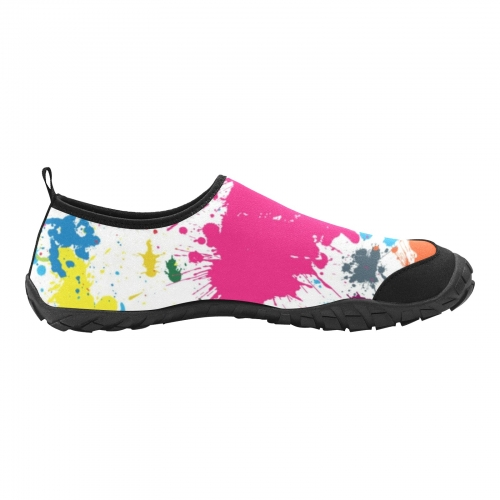•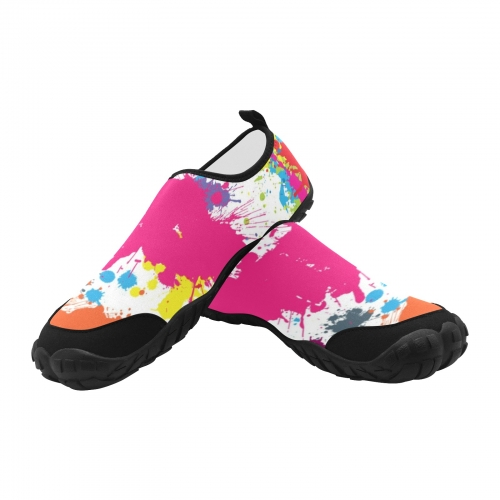•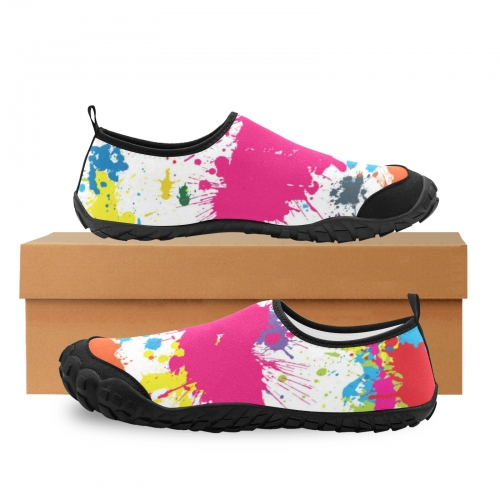### Women's Barefoot Shoes (Model KY21091)

\$30.99
Retail Price \$47.99

Partial Shipping Included

•   Black

EUR36.5=US6=UK3.5=227 mm(L)=8.94 inch(L)=85 mm(W)
EUR37.5=US6.5=UK4=234 mm(L)=9.21 inch(L)=86 mm(W)
EUR38=US7=UK4.5=237 mm(L)=9.33 inch(L)=87 mm(W)
EUR38.5=US7.5=UK5=240 mm(L)=9.45 inch(L)=88 mm(W)
EUR39=US8=UK5.5=243 mm(L)=9.57 inch(L)=89 mm(W)
EUR40=US8.5=UK6=250 mm(L)=9.84 inch(L)=89 mm(W)
EUR40.5=US9=UK6.5=253 mm(L)=9.96 inch(L)=91 mm(W)
EUR41=US9.5=UK7=257 mm(L)=10.12 inch(L)=92 mm(W)
EUR42=US10=UK8=263 mm(L)=10.35 inch(L)=94 mm(W)
EUR42.5=US10.5=UK8.5=267 mm(L)=10.51 inch(L)=96 mm(W)
EUR43=US11=UK9=270 mm(L)=10.63 inch(L)=96 mm(W)
EUR44=US11.5=UK10=276 mm(L)=10.87 inch(L)=100 mm(W)
EUR44.5=US12=UK10.5=279 mm(L)=10.98 inch(L)=101 mm(W)

L=中底长  W=中底宽

Payment methods we accpet: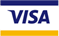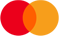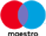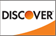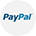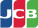We deliver with: